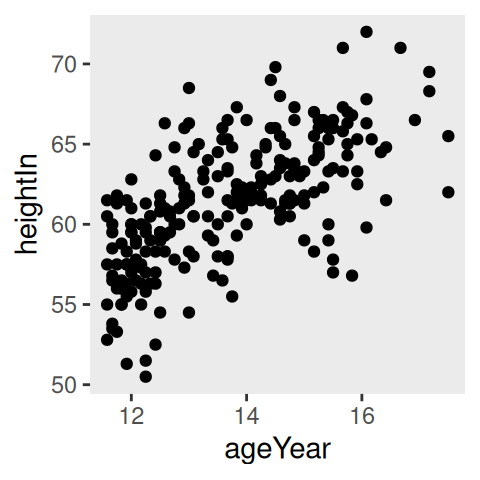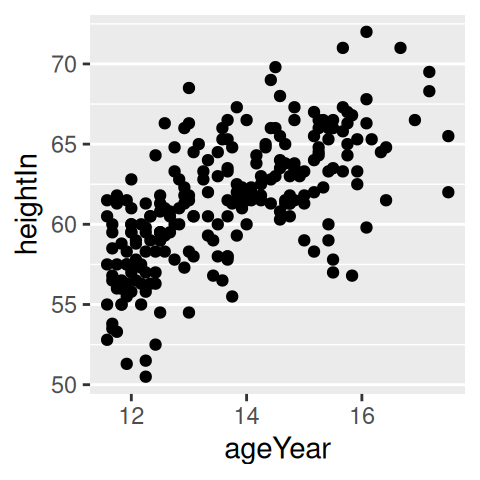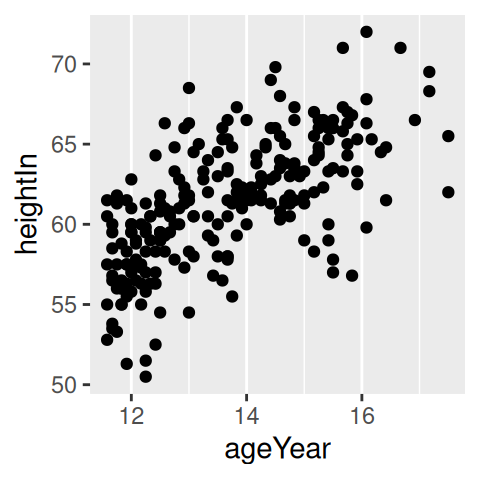## 9.6 Hiding Grid Lines

### 9.6.1 Problem

You want to hide the grid lines in a plot.

### 9.6.2 Solution

The major grid lines (those that align with the tick marks) are controlled with `panel.grid.major`. The minor grid lines (the ones between the major lines) are controlled with `panel.grid.minor`. This will hide them both, as shown in Figure 9.11 (left):

``````library(gcookbook)  # Load gcookbook for the heightweight data set

hw_plot <- ggplot(heightweight, aes(x = ageYear, y = heightIn)) +
geom_point()

hw_plot +
theme(
panel.grid.major = element_blank(),
panel.grid.minor = element_blank()
)``````

### 9.6.3 Discussion

It’s possible to hide just the vertical or horizontal grid lines, as shown in the middle and right-hand graphs in Figure 9.11, with `panel.grid.major.x`, `panel.grid.major.y`, `panel.grid.minor.x`, and `panel.grid.minor.y`:

``````# Hide the vertical grid lines (which intersect with the x-axis)
hw_plot +
theme(
panel.grid.major.x = element_blank(),
panel.grid.minor.x = element_blank()
)

# Hide the horizontal grid lines (which intersect with the y-axis)
hw_plot +
theme(
panel.grid.major.y = element_blank(),
panel.grid.minor.y = element_blank()
)``````Figure 9.11: No grid lines (left); No vertical lines (middle); No horizontal lines (right)